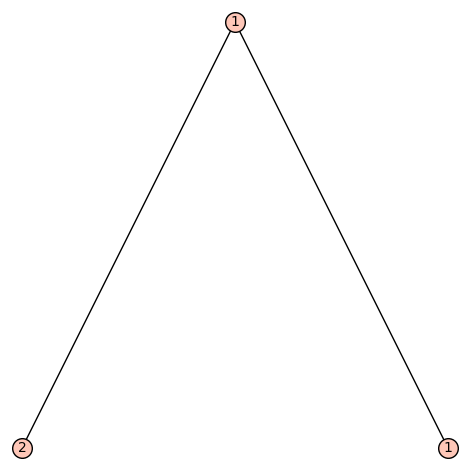# Plotting a LabelledOrderedTree with duplicate labelsI'm attempting to plot a labelled, ordered, rooted tree in Sage using the LabelledOrderedTree class. Here is what I have so far:

sage: n2 = LabelledOrderedTree([], label=2)
sage: n1 = LabelledOrderedTree([], label=1)
sage: root = LabelledOrderedTree([n2, n1], label=1)
sage: root
1[2[], 1[]]


At this point, I've created the tree I'm attempting to build, but here is where I run into problems:

sage: P = root.plot()
...

ValueError: cannot add edge from 1 to 1 in graph without loops


Is there a way to plot a LabelledOrderedTree object when multiple nodes share the same label?

edit retag close merge delete

Sort by » oldest newest most votedThis (kind of silly) limitation is documented as a Warning.

Since you can use arbitrary objects as labels, here's a way around it:

class Wrap(object):
def __init__(self, obj):
self.obj = obj
def __repr__(self):
return repr(self.obj)

n2 = LabelledOrderedTree([], label=Wrap(2))
n1 = LabelledOrderedTree([], label=Wrap(1))
root = LabelledOrderedTree([n2, n1], label=Wrap(1))
root.plot()The two instances Wrap(1) and Wrap(1) are different Python objects, but they look the same, so it works.

Alternatively, if you want new distinct labels:

sage: root.canonical_labelling().plot()

more

Thanks! Hadn't seen that warning, which also states one can alternatively use _latex_(), view, _ascii_art_(), or pretty_print as an alternative to obj.plot() as well. I personally think plot > view in terms of what the end result looks like, but at least it gives me something to work with.